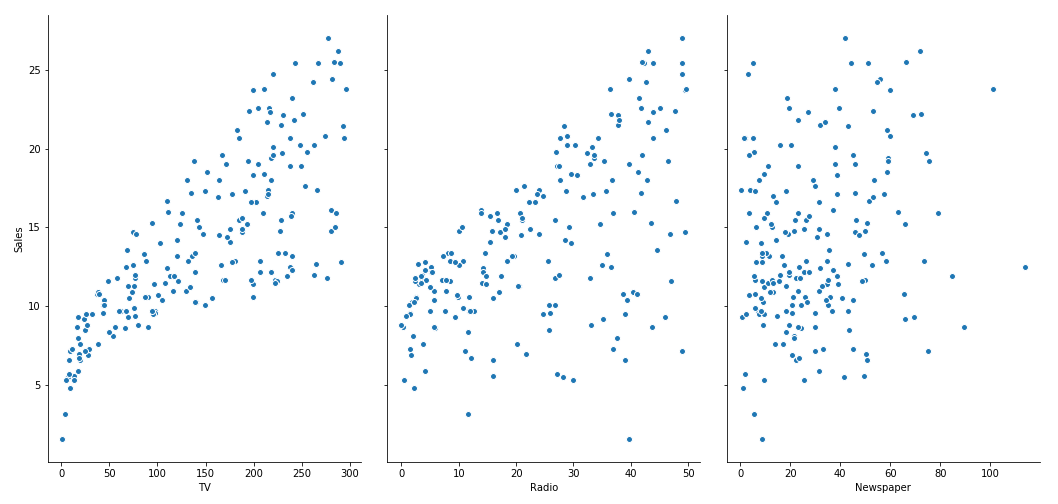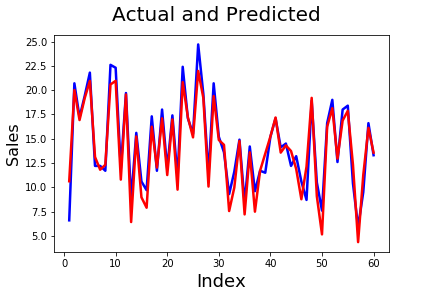## Predict Sales Using Linear Regression: Advertising – Sales Problem

In this problem statement, the manager of a sales team wants to predict sales based on the monthly spending in different media such as TV, newspapers, and Radio. The data set contains monthly spent on TV, Radio, newspapers, and sales. By using multiple linear regression, you can build models using different features. We dive in and see how to predict sales using Linear Regression

#### Step1: Importing and Understanding Data.

```
import pandas as pd
# Looking at the first five rows

Output:

``````	TV 	Radio Newspaper Sales
0 	230.1 	37.8 	69.2 	22.1
1 	44.5 	39.3 	45.1 	10.4
2 	17.2 	45.9 	69.3 	9.3
3 	151.5 	41.3 	58.5 	18.5
4 	180.8 	10.8 	58.4 	12.9``````

#### Step2: Verify values and type of data. This method provides information about dataframe, dtype, and columns.

` advertising_multi.info()`

Output:

``````RangeIndex: 200 entries, 0 to 199
Data columns (total 4 columns):
TV           200 non-null float64
Newspaper    200 non-null float64
Sales        200 non-null float64
dtypes: float64(4)
memory usage: 6.3 KB``````

#### Step3: Let’s look at some statistical information about the dataframe.

This is the way, a user can get some sense about the data if it is completely new to data.

` advertising_multi.describe()`

Output:

``````	TV 	          Radio 	Newspaper 	Sales
count 	200.000000 	200.000000 	200.000000 	200.000000
mean 	147.042500 	23.264000 	30.554000 	14.022500
std 	85.854236 	14.846809 	21.778621 	5.217457
min 	0.700000 	0.000000 	0.300000 	1.600000
25% 	74.375000 	9.975000 	12.750000 	10.375000
50% 	149.750000 	22.900000 	25.750000 	12.900000
75% 	218.825000 	36.525000 	45.100000 	17.400000
max 	296.400000 	49.600000 	114.000000 	27.000000 ``````

#### Step4: Visualising Data.

``` import matplotlib.pyplot as plt
import seaborn as sns
%matplotlib inline

Output:#### Step5: Splitting the Data for dependent and independent attributes.

```
# Putting feature variable to X
# Putting response variable to y

#### Step6 : Split X and y into X_train, X_test, y_train,y_test.

```
from sklearn.cross_validation
import train_test_split
X_train, X_test, y_train, y_test = train_test_split(X, y, train_size=0.7, random_state=100)
```

#### Step7: Performing Linear Regression. Fit the model to the training data.

Here we have used the ‘Scikit’ library to predict sales using linear regression.

```
from sklearn.linear_model import LinearRegression
lm = LinearRegression()
# fit the model to the training data
lm.fit(X_train,y_train)```

#### Step8: Model Evaluation.

```
# print the intercept
print(lm.intercept_)```

Output:

``2.65278966888``

#### Step9: Let’s see the coefficient.

```
coeff_df = pd.DataFrame(lm.coef_, X_test.columns, columns=['Coefficient'])
coeff_df ```

Output:

`````` 	   Coefficient
TV 	   0.045426
Newspaper  0.004603``````

#### Step10: Predict using the model. Test your model with the test dataset.

```
# Making predictions using the model
y_pred = lm.predict(X_test)```

#### Step11: Calculating Error Terms.

```
from sklearn.metrics import mean_squared_error, r2_score
mse = mean_squared_error(y_test, y_pred)
r_squared = r2_score(y_test, y_pred)
print('Mean_Squared_Error :' ,mse)
print('r_square_value :',r_squared)```

Output:

``````Mean_Squared_Error : 1.85068199416
r_square_value : 0.905862210753``````

#### Training using Statsmodels Package:

Statsmodels is a python library that allows performing statistical analysis of data. You can use this to perform linear regression similar to the Scikit-learn library.

```
import statsmodels.api as sm
X_train_sm = X_train

#Unlike Sklearn, statsmodels don't automatically fit a constant,
#so you need to use the method sm.add_constant(X) in order to add a constant.

# Fit the data in one line
lm_1 = sm.OLS(y_train,X_train_sm).fit()

# print the coefficients
lm_1.params```

Output:

``````const        2.652790
TV           0.045426
Newspaper    0.004603
dtype: float64``````

Verify the details of the model.

` print(lm_1.summary())`

Output:

```                           OLS Regression Results
==============================================================================
Dep. Variable:                  Sales   R-squared:                       0.893
Method:                 Least Squares   F-statistic:                     377.6
Date:                Wed, 28 Feb 2018   Prob (F-statistic):           9.97e-66
Time:                        18:17:05   Log-Likelihood:                -280.83
No. Observations:                 140   AIC:                             569.7
Df Residuals:                     136   BIC:                             581.4
Df Model:                           3
Covariance Type:            nonrobust
==============================================================================
coef    std err          t      P&amp;amp;amp;amp;amp;gt;|t|      [0.025      0.975]
------------------------------------------------------------------------------
const          2.6528      0.384      6.906      0.000       1.893       3.412
TV             0.0454      0.002     27.093      0.000       0.042       0.049
Radio          0.1898      0.011     17.009      0.000       0.168       0.212
Newspaper      0.0046      0.008      0.613      0.541      -0.010       0.019
==============================================================================
Omnibus:                       40.095   Durbin-Watson:                   1.862
Prob(Omnibus):                  0.000   Jarque-Bera (JB):               83.622
Skew:                          -1.233   Prob(JB):                     6.94e-19
Kurtosis:                       5.873   Cond. No.                         443.
==============================================================================
```

If you notice at P-value, you can find the P-value of the Newspaper is very high which means the Newspaper attribute is insignificant.

#### Step12: Remove the Newspaper attribute from the dataframe.

```
# Removing Newspaper from our dataframe

# Model building
lm.fit(X_train_new,y_train)

# Making predictions
y_pred_new = lm.predict(X_test_new)```

#### Step13: Now check the R-sq error and RMSE.

```
from sklearn.metrics import mean_squared_error, r2_score

mse = mean_squared_error(y_test, y_pred_new)
r_squared = r2_score(y_test, y_pred_new)

print('Mean_Squared_Error :' ,mse)
print('r_square_value :',r_squared)```

Output:

``````Mean_Squared_Error : 1.78474005209
r_square_value : 0.909216449172``````

#### Step14: Compare actual value vs predicted value.

```#Actual vs Predicted
c = [i for i in range(1,61,1)]
fig = plt.figure()
plt.plot(c,y_test, color="blue", linewidth=2.5, linestyle="-")
plt.plot(c,y_pred, color="red", linewidth=2.5, linestyle="-")
fig.suptitle('Actual and Predicted', fontsize=20) # Plot heading
plt.xlabel('Index', fontsize=18) # X-label
plt.ylabel('Sales', fontsize=16) # Y-label```

Output: